# Bihar Board Class 10th Maths Solutions Chapter 5 Arithmetic Progressions Ex 5.3

Bihar Board Class 10th Maths Solutions Chapter 5 Arithmetic Progressions Ex 5.3 Textbook Questions and Answers.

## BSEB Bihar Board Class 10th Maths Solutions Chapter 5 Arithmetic Progressions Ex 5.3

Question 1.
Find the sum of the following APs:
(i) 2, 7, 12, … , to 10 terms.
(ii) – 37, – 33, – 29, … , to 12 terms.
(iii) 0.6, 1.7, 2.8, … , to 100 terms.
Solution:
(i) Let a be the first term and d be the common difference of the given AP. Then, we have:
a = 2 and d = 7 – 2 = 5
We have to find the sum of 10 terms of the given AP.
Putting a = 2, d = 5 and n = 10 in Sn = $$\frac{n}{2}$$[2a + (n – 1)d], we get
S10 = $$\frac{10}{2}$$ [2 × 2 + (10 – 1)5]
= 5(4 + 9 × 5)
= 5(4 + 45) = 5 × 49 = 245

(ii) Let a be the first term and d be the common difference of the given AP. Then, we have:
a = – 37
and d = – 33 – (- 37) = – 33 + 37 = 4
We have to find the sum of 12 terms of the given AP.
Putting a = – 37, d = 4 and n = 12 in
Sn = $$\frac{n}{2}$$[2a + (n – 1)d] we get,
S12 = $$\frac{12}{2}$$[2 × (- 37) + (12 – 1)4]
= 6(- 74 + 11 × 4)
= 6(- 74 + 44) = 6 × (- 30) = – 180

(iii) Let a be the first term and d be the common difference of the given AP. Then, we have:
a = 0.6 and d = 1.7 – 0.6 = 1.1
We have to find the sum of 100 terms of the given AP.
Putting a = 0.6, d = 1.1 and n = 100 in
Sn = $$\frac{n}{2}$$[2a + (n – 1)d], we get
S100 = $$\frac{100}{2}$$[2 × 0.6 + (100 – 1)1.1]
= 50(1.2 + 99 × 1.1)
= 50(1.2 + 108.9)
= 50 × 110.1
= 5505

(iv) Let a be the first term and d be the common difference of the given AP. Then, we have:
a = $$\frac{1}{15}$$
and d = $$\frac{1}{12}$$ – $$\frac{1}{15}$$ = $$\frac{5-4}{60}$$ = $$\frac{1}{60}$$
We have to find the sum of 11 terms of the given AP.
Putting a = $$\frac{1}{15}$$, d = $$\frac{1}{60}$$ and n = 11 inQuestion 2.
Find the sums given below:
(i) 7 + 10$$\frac{1}{2}$$ + 14 + ………… + 84
(ii) 34 + 32 + 30 + ………… + 10
(iii) – 5 + (- 8) + (- 11) + ………… + (- 230)
Solution:
(i) Here, the last term is given . We will first have to find the number of terms.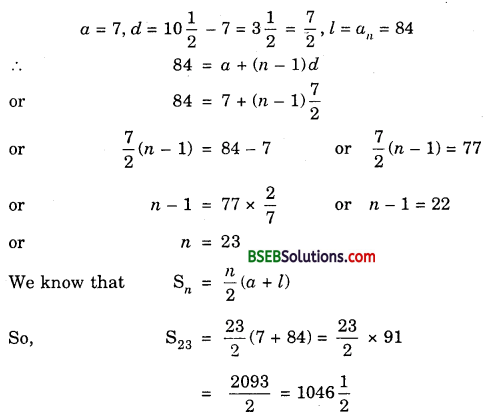(ii) Here, the last term is given. We will first have to find the number of terms.
a = 34, d = 32 – 34 = – 2, l = an = 10
∴ 10 = a + (n – 1)d
or 10 = 34 + (n – 1)(- 2)
or (- 2)(n – 1) = 10 – 34 or (- 2)(n – 1) = – 24
or n – 1 = 12 or n = 12 + 1 = 13
Using Sn = $$\frac{13}{2}$$(34 + 10) = $$\frac{13}{2}$$ × 44
= 13 × 22 = 286

(iii) Here, the last term is given. We will first have to find the number of terms.
a = – 5, d = – 8 – (- 5) = – 8 + 5 = – 3,
l = an = – 230
∴ – 230 = a + (n – 1)d
or – 230 = – 5 + (n – 1)(- 3)
or (- 3)(n – 1) = – 230 + 5
or (- 3)(n – 1) = – 225
or n – 1 = $$\frac{-225}{-3}$$ or n – 1 = 75
or n = 75 + 1 = 76
Using Sn = $$\frac{n}{2}$$(a + l), we have:
S76 = $$\frac{76}{2}$$ (- 5 – 230)
= 38 × (- 235) = – 8930Question 3.
In an AP:
(i) given a = 5, d = 3, an = 50, find n and Sn.
(ii) given a = 7, a13 = 35, find d and S13.
(iii) given a12 = 37, d = 3, find a and S12.
(iv) given a3 = 15, S10 = 125, find d and a10.
(v) given d = 5, S9 = 75, find a and a9.
(vi) given a = 2, d = 8, Sn = 90, find n and an.
(vii) given a = 8, an = 62, Sn = 210, find n and d.
(viii) given an = 4, d = 2, Sn = – 14, find n and a.
(ix) given a = 3, n = 8, S = 192, find d.
(x) given l = 28, S = 144, and there are total 9 terms. Find a.
Solution:
(i) We have:
a = 5, d = 3 and an = 50.
So, a + (n – 1)d = 50 or 5 + (n – 1)3 = 50
or 3(n – 1) = 50 – 5 or n – 1 = $$\frac{45}{3}$$ = 15
or n = 15 + 1 = 16
Putting n = 16, a = 5 and l = an = 50 in Sn = $$\frac{n}{2}$$ (a + l), we get
S16 = $$\frac{16}{2}$$(5 + 50) = 8 × 55 = 440
Hence, n = 16 and S16 = 440.

(ii) We have:
a = 7 and a13 = 35.
Let d be the common difference of the given AP. Then,
a13 = 35 or a + 12d = 35
or 7 + 12d = 35 [∵ a = 7]
or 12d = 35 – 7 = 28 or d = $$\frac{28}{12}$$ = $$\frac{7}{3}$$
Putting n = 13, a = 7 and l = a13 = 35 in Sn = $$\frac{n}{2}$$(a + l), we get
S13 = $$\frac{13}{2}$$(7 + 35) = $$\frac{13}{2}$$ × 42
= 13 × 21 = 273
Hence, d = $$\frac{7}{3}$$ and S13 = 273.

(iii) We have:
a12 = 37 and d = 3.
Let a be the first term of the given AP. Then,
a12 = 37 gives a + 11d = 37
So, a + 11(3) = 37 [∵ d = 3]
or a = 37 – 33 = 4
Putting n = 12, a = 4 and l = a12 = 37 in Sn = $$\frac{n}{2}$$(a + l), we get
S12 = $$\frac{12}{2}$$ (4 + 37) = 6 × 41 = 246
Hence, a = 4 and S12 = 246.

(iv) We have:
a3 = 15 and S10 = 125.
Let a be the first term and d be the common difference of the given AP. Then,
a3 = 15 and S10 = 125
So, a + 2d = 15 ………………… (1)
and $$\frac{10}{2}$$ [2a + (10 – 1)d] = 125
or 5(2a + 9d) = 125
or 2a + 9d = 25 ……………… (2)
2 × (1) – (2) gives
2(a + 2d) – (2a + 9d) = 2 × 15 – 25
or 4d – 9d = 30 – 25
or – 5d = 5 or d = – $$\frac{5}{5}$$ = – 1
Now, a10 = a + 9d = (a + 2d) + 7d
= 15 + 7(- 1) [using (1)]
= 15 – 7 = 8
Hence, d = – 1 and a10 = 8.

(v) We have:
d = 5 and S9 = 75.
Let a be the first term of the given AP. Then,
S9 = 75
So, $$\frac{9}{2}$$ [2a + (9 – 1)5] = 75
or $$\frac{9}{2}$$ (2a + 40) = 75
or 9a + 180 = 75
or 9a = 75 – 180
or 9a = – 105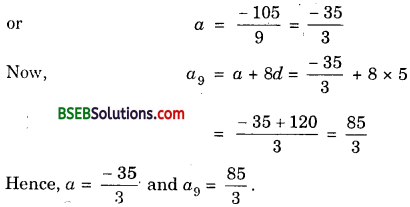(vi) We have:
a = 2, d = 8 and Sn = 90.
Sn = 90 gives
$$\frac{n}{2}$$[2 × 2 + (n – 1)8] = 90
So, $$\frac{n}{2}$$(4 + 8n – 8) = 90
or $$\frac{n}{2}$$(8n – 4) = 90
or n(4n – 2) = 90
or 4n2 – 2n – 90 = 0But n cannot be negative.
∴ n = 5
Now, an = a + (n – 1)d
or a5 = 2 + (5 – 1)8 = 2 + 32 = 34
Hence, n = 5 and an = 34.

(vii) We have:
a = 8, an = 62 and Sn = 210.
Let d be the common difference of the given AP.
Now, Sn = 210
So, $$\frac{n}{2}$$(a + l) = 210
or $$\frac{n}{2}$$(8 + 62) = 210 [∵ a = 8, an = 62]
or $$\frac{n}{2}$$ × 70 = 210
or n = 210 × $$\frac{2}{70}$$ = 3 × 2 = 6
and so an = 62 gives a6 = 62
So, a + 5d = 62
or 8 + 5d = 62 [∵ a = 8]
or 5d = 62 – 8 = 54 or d = $$\frac{54}{5}$$
Hence, d = $$\frac{54}{5}$$ and n = 6.

(viii) We have:
an = 4, d = 2 and Sn = – 14.
Let a be the first term of the given AP. Then,
an = 4 gives
a + (n – 1)2 = 4 [∵ d = 2]
or a = 4 – 2(n – 1) …………. (1)
and Sn = – 14 gives
$$\frac{n}{2}$$(a + l) = – 14 [∵ l = an]
or n(a + 4) = – 28
or n[4 – 2(n – 1) + 41 = – 28
or n(4 – 2n + 2 + 4) = – 28
or n(- 2n + 10) = – 28
or n(- n + 5) = – 14
or – n2 + 5n = – 14
or n2 – 5n + 14 = 0
or (n – 7)(n + 2) = 0
i.e., n = 7 or – 2
But n cannot be negative.
Putting n = 7 in (1), we get
a = 4 – 2(7 – 1) = 4 – 2 × 6
= 4 – 12 = – 8
Hence, n = 7 and a = – 8.

(ix) We have:
a = 3, n – 8 and S = 192.
Let d be the common difference of the given AP.
Sn = $$\frac{n}{2}$$[2a + (n – 1)d] gives
192 = $$\frac{8}{2}$$ [2 × 3 + (8 – 1)d]
or 192 = 4(6 + 7d) or 48 = 6 + 7d
or 7d = 48 – 6 or 7d = 42
or d = $$\frac{42}{7}$$ = 6
Hence, d = 6

(x) We have:
l = 28, S = 144 and n = 9.
Let a be the first term of the given AP.
S = 144 gives $$\frac{n}{2}$$(a + l) = 144
or $$\frac{9}{2}$$(a + 28) = 144 or a + 28 = 144 × $$\frac{2}{9}$$
or a + 28 = 32 or a = 32 – 28 = 4
Hence, a = 4.Question 4.
How many terms of the AP: 9, 17, 25, … must be taken to give a sum of 636?
Solution:
Let the first term be a = 9 and common difference be d = 17 – 9 = 8.
Let the sum of n terms be 636. Then,
Sn = 636
So, $$\frac{n}{2}$$[2a + (n – 1)d] = 636
or $$\frac{n}{2}$$[2 × 9 + (n – 1)d] = 636
or $$\frac{n}{2}$$(18 + 8n – 8) = 636
or $$\frac{n}{2}$$(8n + 10) = 636
or n(4n + 5) = 636 or 4n2 + 5n – 636 = 0But n cannot be negative.
∴ n = 12.
Thus, the sum of 12 terms is 636.

Question 5.
The first term of an AP is 5, the last term is 45 and the sum is 400. Find the number of terms and the common difference.
Solution:
Let a be the first term and d be the common difference of the AP such that
a = 5, l = 45 and S = 400
∴ S = 400 gives $$\frac{n}{2}$$(a + l) = 400
or n(5 + 45) = 400 × 2 or n(50) = 400 × 2
or n = $$\frac{400×2}{50}$$ = 8 × 2 = 16
Also, l = 45
So, a + (n – 1)d = 45
or 5 + (16 – 1)d = 45
or 15d = 45 – 5 = 40 or d = $$\frac{40}{15}$$ = Hence, the number of terms is 16 and the common difference is $$\frac{8}{3}$$.

Question 6.
The first and the last terms of an AP are 17 and 350 respectively. If the common difference is 9, how many terms are there and what is their sum?
Solution:
Let a be the first term and d be the common difference. Let l be its last term. Then, a – 17, l = an = 350 and d = 9.
l = an = 350 gives
a + (n – 1)d = 350
or 17 + (n – 1)9 = 350
or 9(n – 1) = 350 – 17 = 333
or n – 1 = $$\frac{333}{9}$$ = 37 or n = 37 + 1 = 38
Putting a = 17, l = 350 and n = 38 in Sn = $$\frac{n}{2}$$(a + l), we get
S38 = $$\frac{38}{2}$$(17 + 350)
= 19 × 367 = 6973
Hence, there are 38 terms in the AP having their sum as 6973.Question 7.
Find the sum of first 22 terms of an AP in which d = 7 and 22nd term is 149.
Solution:
Let a be the first term and d be the common difference of the given AP. Then,
d = 7 and a22 = 149
So, a + (22 – 1)d = 149 or a + 21 × 7 = 149
or a = 149 – 147 = 2
Putting n = 22, a – 2 and d = 7 in Sn = $$\frac{n}{2}$$[2a + (n – 1)d], we get
S22 = $$\frac{22}{2}$$[2 × 2 + (22 – 1)7]
= 11(4 + 21 × 7) = 11(4 + 147)
= 11 × 151 = 1661
Hence, the sum of first 22 terms of the AP is 1661.

Question 8.
Find the sum of first 51 terms of an AP whose second and third terms are 14 and 18 respectively.
Solution:
Let a be the first term and d be the common difference of the given AP. Then,
a2 = 14 and a3 = 18
So, a + d = 14 and a + 2d = 18
Solving these equations, we get
d = 4 and a = 10 Putting a = 10,
d = 4 and n = 51 in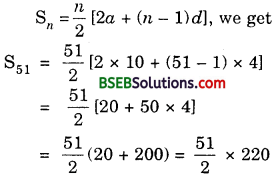= 51 × 110 = 5610

Question 9.
If the sum of 7 terms of an AP is 49 and that of 17 terms is 289, find the sum of n terms.
Solution:
Let a be the first term and d be the common difference of the given AP. Then,
S7 = 49 and S17 = 289Solving these two equations, we get
d = 2 and a = 1Question 10.
Show that a1, a2, …, an, … form an AP where an is defined as below:
(i) an = 3 + 4n
(ii) an = 9 – 5n
Also, find the sum of the first 15 terms in each case.
Solution:
(i) We have:
an = 3 + 4n
Substituting n = 1, 2, 3, 4, …………. n, we get
The sequence 7, 11, 15, 19, …, (3 + 4n), which is an AP with common difference 4.
Putting a = 7, d = 4 and n = 15 in Sn = $$\frac{n}{2}$$[2a + (n – 1)d], we get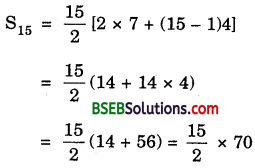= 15 × 35 = 525

(ii) We have:
an = 9 – 5n
Substituting n = 1, 2, 3, 4, n, we get the sequence 4, – 1, – 6, – 11, …, (9 – 5n), which is an AP with common difference – 5.
Putting a = 4, d = – 5 and n = 15 inQuestion 11.
If the sum of the first n terms of an AP is 4n – n2, what is the first term (that is S1)? What is the sum of first two terms? What is the second term? Similarly, find the 3rd, the 10th and the nth terms.
Solution:
According to the question,
Sn = 4n – n2.
∴ S1 = 4 × 1 – 12 = 4 – 1 = 3
Thus, First term = 3
Now, sum of first two terms = S2 = 4 × 2 – 22
= 8 – 4 = 4
∴ Second term = S2 – S1 = 4 – 3 = 1
S3 = 4 × 3 – 32 = 12 – 9 = 3
∴ Third term = S3 – S2 = 3 – 4 = – 1
S3 = 4 × 3 – 32
= 36 – 81
= – 45
and S10 = 4 × 10 – 102
= 40 – 100 = – 60
∴ Tenth term = S10 – S9
= – 60 – (- 45)
= – 60 + 45 = – 15
Also, Sn = 4n – n2
and Sn-1 = 4(n – 1) – (n – 1)2
= 4n – 4 – n2 + 2n – 1
= – n2 + 6n – 5
∴ nth term = Sn – Sn-1
= 4n – n2 – (- n2 + 6n – 5)
= 4n – n2 + n2 – 6n + 5
= 5 – 2n

Question 12.
Find the sum of the first 40 positive integers divisible by 6.
Solution:
The first 40 positive integers divisible by 6 are 6, 12, 18, ….. Clearly, it is an AP with first term a = 6 and common difference d = 6. We want to find S40.
∴ S40 = $$\frac{40}{2}$$ [2 × 6 + (40 – 1)6]
= 20(12 + 39 × 6)
= 20(12 + 234) = 20 × 246 = 4920

Question 13.
Find the sum of the first 15 multiples of 8.
Solution:
The first 15 multiples of 8 are 8 × 1, 8 × 2, 8 × 3, …………., 8 × 15
i.e., 8, 16, 24, …, 120, which is an AP.
∴ Sum of 1st 15 multiples of 8 = $$\frac{15}{2}$$(8 + 120)
[Sn = $$\frac{n}{2}$$(a + l)]
= $$\frac{15}{2}$$ × 128
= 15 × 64 = 960Question 14.
Find the sum of all the odd numbers between 0 and 50.
Solution:
The odd numbers between 0 and 50 are 1, 3, 5, …, 49. They form an AP and there are 25 terms.
∴ Their sum = $$\frac{25}{2}$$ (1 + 49) = $$\frac{25}{2}$$ × 50
= 25 × 25 = 625

Question 15.
A contract on construction job specifies a penalty for delay of completion beyond a certain date as follows: Rs 200 for the first day, Rs 250 for the second day, Rs 300 for the third day, etc., the penalty for each succeeding day being Rs 50 more than for the preceding day.
How much money the contractor has to pay as penalty, if he has delayed the work by 30 days?
Solution:
Here, a = 200, d = 50 and n = 30.
∴ S = $$\frac{30}{2}$$[2 × 200 + (30 – 1)50]
[∵ Sn = $$\frac{n}{2}$$(2a + (n – 1)d]
= 15(400 + 29 × 50)
= 15(400 + 1450)
= 15 × 1850
= 27750
Hence, a delay of 30 days costs the contractor Rs 27750.

Question 16.
A sum of Rs 700 is to be used to give seven cash prizes to students of a school for their overall academic performance. If each prize is Rs 20 less than its preceding prize, find the value of each of the prizes.
Solution:
Let the respective prizes be a + 60, a + 40, a + 20, a, a – 20, a – 40, a – 60
∴ The sum of the prizes is
a + 60 + a + 40 + a + 20 + a + a – 20 + a – 40 + a – 60 = 700
or 7a = 700 or a = $$\frac{700}{7}$$ = 100
∴ The seven prizes are 100 + 60, 100 + 40, 100 + 20, 100, 100 – 20, 100 – 40, 100 – 60, i.e., 160, 140, 120, 100, 80, 60, 40 (in Rs).

Question 17.
In a school, students thought of planting trees in and around the school to reduce air pollution. It was decided that the number of trees, that each section of each class will plant, will be the same as the class, in which they are studying e.g., a section of Class I will plant 1 tree, a section of Class II will plant 2 trees, and
so on till Class XII. There are three sections of each class. How many trees will be planted by the students?
Solution:
Since there are three sections of each class, so the number of trees planted by class I, class II, class III, …, class XII are 1 × 3, 2 × 3, 3 × 3, ………….., 12 × 3 respectively.
i.e., 3, 6, 9, …, 36. Clearly, it forms an AP.
The sum of the number of the trees planted by these classes
= $$\frac{12}{2}$$(3 + 36) = 6 × 39 = 234Question 18.
A spiral is made up of successive semicircles, with centres alternately at A and B, starting with centre at A, of radii 0.5 cm, 1.0 cm, 1.5 cm,
2.0 cm, … as shown in fig. What is the total length of such a spiral made up of thirteen consecutive semicircles? (Take π = $$\frac{22}{7}$$)Solution:
Length of a semi-circumference = πr, where r is the radius of the circle.
∴ Length of spiral made up of thirteen consecutive semicircles
= (π × 0.5 + π × 1.0 + π × 1.5 + π × 2.0 + … + π × 6.5) cm
= π × 0.5(1 + 2 + 3 + … + 13) cm
= π × 0.5 × $$\frac{13}{2}$$(2 × 1 + [13 – 1) × 1] cm
= $$\frac{22}{7}$$ × $$\frac{5}{10}$$ × $$\frac{13}{2}$$ × 14 cm = 143 cm

Question 19.
200 logs are stacked in the following manner. 20 logs in the bottom row, 19 in the next row, 18 in the row next to it, and so on (see figure).
In how many rows are the 200 logs placed and how many logs are in the top row?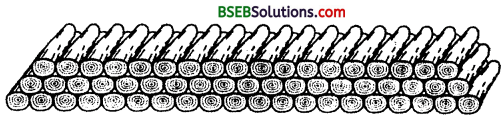Solution:
Clearly, logs stacked in each row form a sequence 20, 19, 18, 17, …. It is an AP with a = 20 and d = 19 – 20 = – 1.
Let Sn = 200. Then,
$$\frac{n}{2}$$[2 × 20 + (n – 1)(- 1)] = 200
or n(40 – n + 1) = 400
or n2 – 41n + 400 = 0
or (n – 16)(n – 25) = 0
i.e., n = 16 or 25
Here, the common difference is negative.
The terms go on diminishing and 21st term becomes zero. All terms after 21st term are negative.
These negative terms when added to positive terms from 17th term to 20th term, cancel out each other and the sum remains the same.
Thus, n = 25 is not valid for this problem. So, we take n = 16
Thus, 200 logs are placed in 16 rows.
Number of logs in the 16th row = a16
= a + 15d
= 20 + 15(- 1)
= 20 – 15 = 5Question 20.
In a potato race, a bucket is placed at the starting point, which is 5 m from the first potato, and the other potatoes are placed 3 m apart in a straight line.
There are ten potatoes in the line (see figute below).A competitor starts from the bucket, picks up the nearest potato, runs back with it, drops it in the bucket, runs back to pick up the next potato, runs to the bucket to drop it in, and she continues in the same way until
all the potatoes are in the bucket. What is the total distance the competitor has to run?
Solution:
To pick up the first potato, second potato, third potato, fourth potato, …………,
the distances (in metres) run by the competitor are
2 × 5; 2 × (5 + 3), 2 × (5 + 3 + 3), 2 × (5 + 3 + 3 + 3), …
i.e., 10, 16, 22, 28, …
which is in AP with a = 10 and d = 16 – 10 = 6.
The sum of first ten terms,
S10 = $$\frac{10}{2}$$ [(2 × 10 + (10 – 1) × 6)]
= 5(20 + 54)
= 5 × 74
= 370
∴ The total distance the competitor has to run is 370 m.